## Example Questions

2 Next →

### Example Question #11 : Sat Mathematics

Assume l and m are parallel horizontal lines where l is above m. A third straight line k intersects both lines l and m, creating a total of 4 different angles on each of the two intersections. The intersections of lines l and m contain angles a, b, c, d and w, x, y, z, respectively. The angle layouts start with a and w in the top left position with the other angles following in alphabetical order in the clockwise direction. What is the degree value of angle z if angle c has a value of 67 degrees?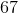degrees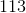degrees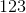degreesdegreesdegreesdegrees

Explanation:

The purpose of this question is to understand the process of using geometry to find degree values of angles.

Since l and m are parallel lines, the respective angles on both lines will have equivalent degree values. This means that a and w, b and x, and so on will be the same degree amount. A straight line is 180 degrees, so you know that angle d is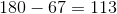degrees; and therefore angle z, which has the same value, is also 113 degrees.

### Example Question #11 : Plane Geometry

Angle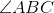measures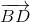is the bisector of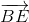is the bisector ofWhat is the measure of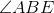?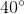Explanation:Let's begin by observing the larger angle.is cut into two 10-degree angles by. This means that anglesand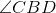equal 10 degrees. Next, we are told thatbisects, which creates two 5-degree angles.consists of, which is 10 degrees, and, which is 5 degrees. We need to add the two angles together to solve the problem.2 Next →

### All SAT Math Resources